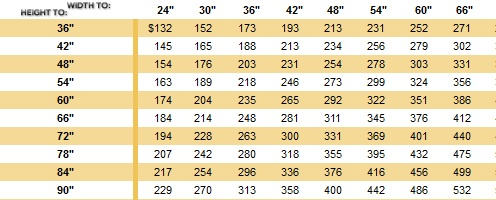# PHP Convert CSV Price Matrix to MySQL Table

Recently, I had needed to convert a CSV file that was based on a price matrix based a width by height chart. The products had different size increments for width and height and products. I needed to come up with a solution for making this work dynamically.

The price matrix works by finding the column of the closest width (x) without going over, then finding the closest height (y) without going over. Then where coordinates (x,y) intersect reads a price. The price matrix below shows about a 1/3 of the actual table.In order to easily create the CSV file was by a handy little trick using Excel. The table above is from a web page, so by copying the HTML table into its own file, and then save with a .xls extension. Open the Excel file, and then save file as a CSV file.  This was a little bit of clicking, but this is an extemely easy way to create the CSV file with the price matrix to convert to MySQL.

Now the that CSV was created, I needed a way to parse the file in PHP to get both the width and height columns and corresponding price.  I knew using some of the built in functions like fgetscsv is fine for just needing rows of data. I did some searches on some forums I frequent, and found CSV Library. It was written by Jelmer, an active member, and was being used with CodeIgniter.  The library is a simple class wrapper with few handy features that made this all work. There were some built in methods that allowed to get column names, remove colums, and manipulate the content.

Now for some the code to make this all work:

```1 2 3 4 5 6 7 8 //include CSV Reader class require_once('CSVReader.php');   //create CSV object \$csv = Csv::get('pricing.csv');   //store CSV contents \$cells = \$csv->get_contents();```

Cell contents is now available, we need to get the width column and the row with the heights.

```9 10 11 12 13 14 15 16 17 18 19 20 21 22 23 24 //Get height column before removing it from cells for (\$i = 0;\$i < count(\$cells);\$i++) { \$columns[] = \$cells[\$i]; }   //remove the first column from every row since these are our height increments \$csv->remove_column(0);   //get cell contents again \$cells = \$csv->get_contents();   //get the row of width increments \$stack = \$csv->field_names;   //remove first blank cell in the height row because this was a placeholder \$field_names = array_shift(\$stack);```

At this point we have the width increments, height increments, and cell contents (prices) in arrays. Next we need to loop through the cells of prices in the matrix to find the respective width and height.

```25 26 27 28 29 30 31 32 33 34 //Put it back together //loop through each row for (\$i = 0;\$i < count(\$cells); \$i++) { //loop through each column to pair row index with height index with price for (\$j = 0;\$j < count(\$cells[\$i]);\$j++) { echo "INSERT INTO frames (width,height,price) VALUES('" . str_replace('"', '', trim(\$stack[\$j]))."','" . str_replace('"', '', trim(\$columns[\$i])) . "','" . \$cells[\$i][\$j+1] ."');". "<br/>\n"; } }```

This echoes out some sql statments that you would want to import into your MySQL table. So, now we have converted the price matrix HTML table to a CSV file, and have imported it into MySQL. Now we need to use the database table to lookup a price. This is very easy and straightforward to do with one simple query.

This is how the table looks

```+-------+--------+-------+ | width | height | price | +-------+--------+-------+ | 24 | 36 | 132 | | 24 | 42 | 145 | | 24 | 48 | 154 | | 24 | 54 | 163 | | 24 | 60 | 174 | | 24 | 66 | 184 | | 24 | 72 | 194 | | 24 | 78 | 207 | | 24 | 84 | 217 | | 24 | 90 | 229 | +-------+--------+-------+```

Say we wanted to find the price for a frame that is 36″ x 60″. We would use this query below:

`SELECT price FROM frames WHERE width >= 36 AND height >= 60 ORDER BY height, width LIMIT 1;`

This will return the result 235, and if you look in the example price matrix grid above you will see the same number. The query works by finding all rows as big or bigger than the given size. The ORDER BY clause will sort the results from smallest to largest. Since we want the smallest possible step in the matrix the LIMIT 1, retrieves the first row.